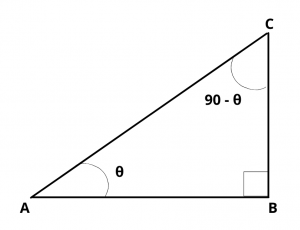# Prove that $$Cot(90 – \theta)$$ = $$Tan\theta$$.

## Solution :

Draw a right angled triangle ABC right angled at B.Let $$\angle$$ A = $$\theta$$,  then $$\angle$$ C = 90 – $$\theta$$

tan A = $$tan\theta$$ = $$BC\over AB$$                     ……..(1)

cot C = $$cot(90 – \theta)$$ = $$BC\over AB$$             ……..(2)

From (1) and (2), we have

$$cot(90 – \theta)$$ = $$tan\theta$$